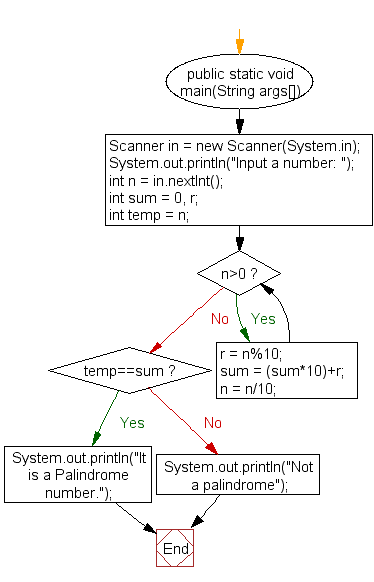﻿ Java exercises: Check if a number is palindrome or not - w3resource# Java Exercises: Check if a number is palindrome or not

## Java Numbers: Exercise-24 with Solution

Write a Java program to check if a number is palindrome or not.

In number system a palindromic number is a number that is the same when written forwards or backwards, i.e., of the form.
The first few palindromic numbers are therefore are 0, 1, 2, 3, 4, 5, 6, 7, 8, 9, 11, 22, 33, 44, 55, 66, 77, 88, 99, 101, 111, …

Test Data
Input a number: 5

Pictorial Presentation:Sample Solution:

Java Code:

``````import java.util.Scanner;
public class Example24  {

public static void main(String args[])
{
Scanner in = new Scanner(System.in);
System.out.print("Input a number: ");
int n = in.nextInt();
int sum = 0, r;
int temp = n;
while(n>0)
{
r = n % 10;
sum = (sum*10)+r;
n = n/10;
}
if(temp==sum)
System.out.println("It is a Palindrome number.");
else
System.out.println("Not a palindrome");
}
}
```
```

Sample Output:

```Input a number: 5
It is a Palindrome number.
```

Flowchart:Java Code Editor:

What is the difficulty level of this exercise?

Test your Programming skills with w3resource's quiz.

﻿

```str1.toLowerCase().contains(str2.toLowerCase())